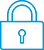cancel
Showing results for
Did you mean:It has been a while since anyone has replied. Simply ask a new question if you would like to start the discussion again.Level 1
15 9 0 0
Message 1 of 6
1,379
Flag Post

Solved!

# Exasperater with HP Prime Graphing CalculatorHP Recommended
HP Prime Graphing Calculator

I'm exasperated. The HP Prime Graphing Calculator I purchased 2 weeks ago sucks. One shouldn't have to fight the calculator to get it to do what the User Guide (UG) says it will do. Can you imagine fighting your calculator in an AP test?

1. The UG says it will divide polynomials symbolically. Doesn't work, but it will divide them if they are presented as lists of coefficients.
2. CONVERT (123,base,8) doesn't work as described in the UG. But if you spell out the command out in lowercase it works.
3. dsolve(), the command to solve a differential equation doesn't work unless you edit the command. deSolve() works.
4. The Function app is described in the UG , beginning on page 109. It mostly works. However, page 129, the section called "Defining functions in terms of derivatives and Integrals," is the point of distress. The example works as advertised: F1(x) is the function, F2(x) is the derivative, and F3(x) is the integral. The Plot is correct. Change F1(x) to cos(x) and display the plot. It's also correct: F1 is cos, F2 is -sin, and F3 is sin. No problem. But change F1 to sin(x) and plot. F1 is sin ... correct. F2 is cos ... correct F3 is (-cos + 1), -cos shifted up by 1 ... incorrect. Suggests there's a problem with the calculator. But using the Connectivity Kit, the latest is version 20181016, which is the version in my calculator.
5. There's a "Virtual Calculator" pdf file in the list of downloads on the web sigt. But where's the exe file?

What's the best ad for a TI graphing calculator? So far it seems to be the HP Prime Graphing Calculator.

Tags (1)
1 ACCEPTED SOLUTION

Accepted SolutionsLevel 9
3,551 3,458 180 540
Message 2 of 6
Flag PostHP Recommended

Hi!, @Bob931 :

For User Guide in the Emulator, you needed press in Help. Then appear …

Quick Start Guide

User Guide

etc ...

For convert units ...Kind Regards !.
Have a nice day !.
Provost in HP Spanish Public Forum ... https://h30467.www3.hp.com/
Tags (1)
5 REPLIES 5Level 9
3,551 3,458 180 540
Message 2 of 6
Flag PostHP Recommended

Hi!, @Bob931 :

For User Guide in the Emulator, you needed press in Help. Then appear …

Quick Start Guide

User Guide

etc ...

For convert units ...Kind Regards !.
Have a nice day !.
Provost in HP Spanish Public Forum ... https://h30467.www3.hp.com/
Tags (1)Level 1
15 9 0 0
Message 3 of 6
Flag PostHP Recommended

I believe my calculator is defective. Is yours?

Function app. Home view.

Set up 2 functions in the Symbolic view.

Press "Symb" key, above "Plot"

Set F1(x) = sin(x)

Set F2(x) = {integral sign with lower limit 0, upper limit x, integrand is F1(t)dt} as shown in the User Guide under "Functions defined as integrals," page 132 (182 of 761).

Press "Plot." The plot is incorrect. F1 is sin. F2 is (-cos +1). But if you change F1 to cos(x) and re-plot the plot is correct, F1 is cos, F2 is sin.

Is your calculator as defective as mine?Level 8
649 634 124 222
Message 4 of 6
Flag PostHP Recommended

But the integral of sin(t), dt, from 0 to x, is in fact equal to 1-cos(x), which is what the HP Prime says it is. The HP 50g returns the same result, as does Wolfram Alpha. Why do you consider it incorrect?

-Joe-Level 1
15 9 0 0
Message 5 of 6
Flag PostHP Recommended

Thanks for correcting me.

I was thinking of the integral of sin as -cos.

The example isn't of the indefinite integral, but of the "definite" integral from 0 to X.

So. I yield, you are correct, and my calculator is not defective, I am.

Thanks.Level 1
15 9 0 0
Message 6 of 6
Flag PostHP Recommended

Thanks for the info. I'm not sure we're on the same page. I don't understand your '"Emulator" reference. I'm using my calculator.

Function app. CAS mode.

If you get the CONVERT command from the toolbox Catalog it's in all CAPS.

My calculator gives "bad argument error" if I use the All CA PS command.

CONVERT(123,base,8) gives bad argument error when I press Enter.

But if I type the command in lower case it gives the expected result.

convert(123,base,8)  gives [3 7 1]  Notice no commas.

† The opinions expressed above are the personal opinions of the authors, not of HP. By using this site, you accept the Terms of Use and Rules of Participation